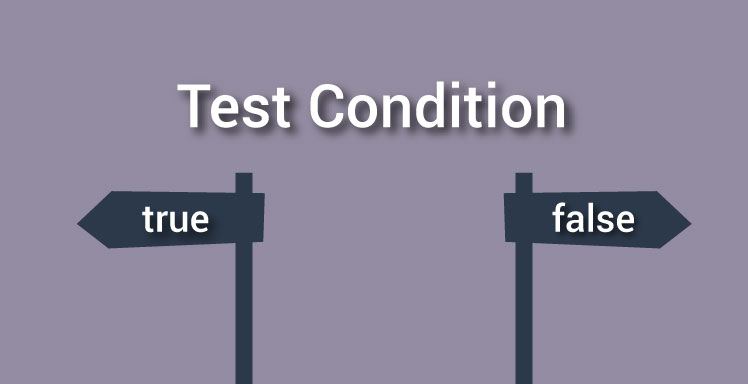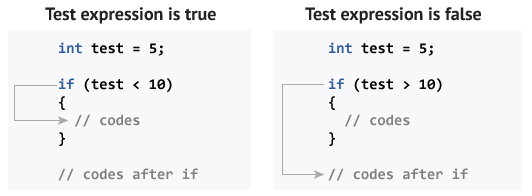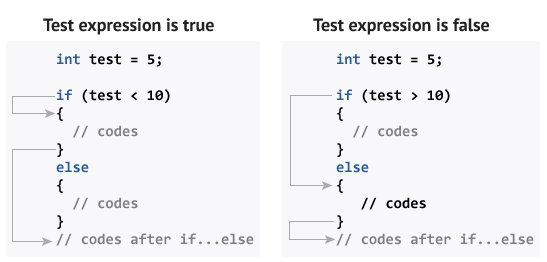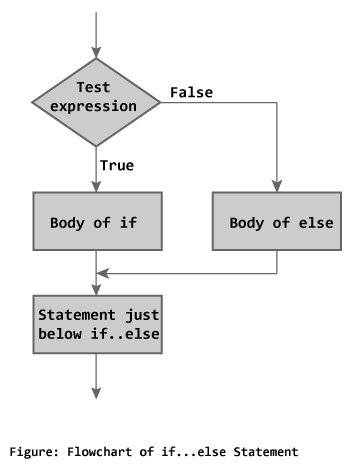# C++ if, if...else and Nested if...else

In this article, you will learn to create decision making statements in a C++ program using different forms of if..else statement.Contents
if Statement
if...else Statement
Nested if...else Statement
Conditional Operator

## C++ if Statement

```if (testExpression)
{
// statements
}```

The `if` statement evaluates the test expression inside parenthesis.

If test expression is evaluated to true, statements inside the body of `if` is executed.

If test expression is evaluated to false, statements inside the body of `if` is skipped.

### How if statement works?### Flowchart of if StatementAbove figure describes the working of an if statement.

### Example 1: C++ if Statement

``````// Program to print positive number entered by the user
// If user enters negative number, it is skipped

#include <iostream>
using namespace std;

int main()
{
int number;
cout << "Enter an integer: ";
cin >> number;

// checks if the number is positive
if ( number > 0)
{
cout << "You entered a positive integer: " << number << endl;
}

cout << "This statement is always executed.";
return 0;

}
``````

Output 1

```Enter an integer: 5
You entered a positive number: 5
This statement is always executed.```

Output 2

```Enter a number: -5
This statement is always executed.```

## C++ if...else

The `if else` executes the codes inside the body of `if` statement if the test expression is true and skips the codes inside the body of `else`.

If the test expression is false, it executes the codes inside the body of `else` statement and skips the codes inside the body of `if`.

### How if...else statement works?### Flowchart of if...else### Example 2: C++ if...else Statement

``````// Program to check whether an integer is positive or negative
// This program considers 0 as positive number

#include <iostream>
using namespace std;

int main()
{
int number;
cout << "Enter an integer: ";
cin >> number;

if ( number >= 0)
{
cout << "You entered a positive integer: " << number << endl;
}

else
{
cout << "You entered a negative integer: " << number << endl;
}

cout << "This line is always printed.";
return 0;
}``````

Output

```Enter an integer: -4
You entered a negative integer: -4.
This line is always printed.
```

## C++ Nested if...else

The `if...else` statement executes two different codes depending upon whether the test expression is true or false. Sometimes, a choice has to be made from more than 2 possibilities.

The nested `if...else` statement allows you to check for multiple test expressions and execute different codes for more than two conditions.

### Syntax of Nested if...else

```if (testExpression1)
{
// statements to be executed if testExpression1 is true
}
else if(testExpression2)
{
// statements to be executed if testExpression1 is false and testExpression2 is true
}
else if (testExpression 3)
{
// statements to be executed if testExpression1 and testExpression2 is false and testExpression3 is true
}
.
.
else
{
// statements to be executed if all test expressions are false
}```

### Example 3: C++ Nested if...else

``````// Program to check whether an integer is positive, negative or zero

#include <iostream>
using namespace std;

int main()
{
int number;
cout << "Enter an integer: ";
cin >> number;

if ( number > 0)
{
cout << "You entered a positive integer: " << number << endl;
}
else if (number < 0)
{
cout<<"You entered a negative integer: " << number << endl;
}
else
{
cout << "You entered 0." << endl;
}

cout << "This line is always printed.";
return 0;
}``````

Output

```Enter an integer: 0
You entered 0.
This line is always printed.```

## Conditional/Ternary Operator ?:

A ternary operator operates on 3 operands which can be used instead of a `if...else` statement.

Consider this code:

```if ( a < b ) {
a = b;
}
else {
a = -b;
}
```

You can replace the above code with:

```a = (a < b) ? b : -b;
```

The ternary operator is more readable than a `if...else` statement for short conditions.